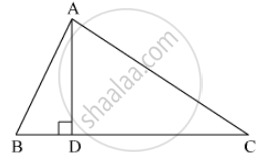# In ∆ Abc, Ad ⊥ Bc. Prove That Ac2 = Ab2 +Bc2 − 2bc X Bd - Mathematics

Sum

In ∆ ABC, AD ⊥ BC.
Prove that  AC2 = AB2 +BC2 − 2BC x BDIn ∆ ABC (Figure 3), AD ⊥ BC.
Prove that  AC2 = AB2 +BC2 − 2BC x BD#### Solution

Applying Pythagoras theorem in ΔADB, we obtain

⇒ AD2 = AB2 − DB2                                               .....(1)

Applying Pythagoras theorem in ΔADC, we obtain

AB2 − BD2 + DC2 = AC2    ...[Using equation (1)]

AB2 − BD2 + (BC − BD)2 = AC2

AC2 = AB2 − BD2 + BC2 + BD2 −2BC x BD

AC2 = AB2 + BC2 − 2BC x BD

Concept: Right-angled Triangles and Pythagoras Property
Is there an error in this question or solution?